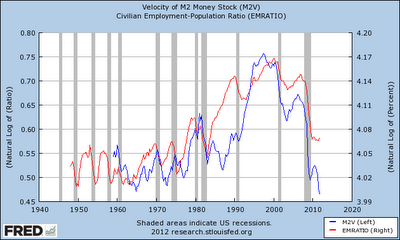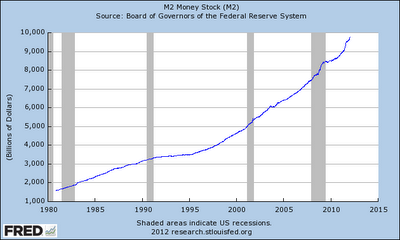### Graph(s) of the week: velocity of money

Still on the US business cycle, here is the look at some monetary indicators. The first graph depicts the velocity of money (V in the famous quantitative theory of money equation MV=PQ) and the employment-population ratio (employment adjusted for the discouraged workforce - the most precise unemployment indicator):Source: St. Louis Fed database (FRED)
The natural log of M2 velocity is depicted on the left scale (blue), while the log of the E-P ratio is on the right scale (red). The correlation is obvious and can be explained in the following way: the velocity of money measures the rate of circulation of money in the economy, i.e. the rate at which goods and services are bought. So it makes sense that with decreasing levels of employment and decreasing consumer confidence (as seen in the previous post), the rate of money used to buy goods or services will decrease. And since the E-P ratio still isn't showing any signs of improvement (even with the latest jobs report), the velocity of money is still decreasing without showing any positive signs so far. (Note: the M2 velocity is calculated by dividing the nominal GNP with the money supply M2).

On a different note, to further examine the quantitative theory of money in the current situation, here is a graph depicting the M2 money supply for the US:Source: St. Louis Fed database (FRED)
Its steady increase, multiplied by the velocity decrease and divided by the stagnant nominal GNP can explain why the price level hasn't erupted due to a large level of QE. The thing pondering many economists is whether this inflation will pick up once the velocity of money rises with the recovery. Perhaps this can shed new light on the ultimate determinants of inflation particularly in times of crises, as it seems that the velocity of money can explain inflation better than the growth rate of the money supply. But this is just a though.
One thing is certain though, parameter V isn't constant as assumed by the QTM - it is clearly highly volatile and sensitive to the aggregate demand shocks.

1.So, if the velocity is calculated by dividing NGNP with the money supply, an increase of the money supply will decrease the velocity. Isn’t this happening in the last two years? More money in the economy decreased its velocity, particularly since banks are still holding on to a lot of that money.

1.But over time the price of the whole gdp goes up with money supply. Plot it logrithmicly.

So, all that we make, we should be able to buy but all of it cost more.

Why? NGNP and money supply tend to go up roughly exponentially compared to velocity which comaritively goes no where compared to an exponential.

If you want to be fair if the money supply did not go up and production goes up over time we should get more for our money. Our share of our greater production.

Here is the graph. The distance between the GDP and money supply line is the log of velocity. Remember ln(g/m)=ln(g)-ln(m), a difference. You might remember ln(a*b)=ln(a) + ln(b) first.

http://research.stlouisfed.org/fred2/graph/?g=HcQ

2.Exactly, if you have more money in the economy, it will take it longer to circulate, and thus lower the velocity parameter. But as you may see from the bottom graph, money supply is increasing at pretty much constant rates for the past 30 years, implying that its velocity was pretty much dependent on the nominal GNP. And during the crisis in particular; GNP has been hit, M2 was increasing, resulting in a lower level of V. This is why velocity of money can be a good indicator of economic activity – even though the ratio itself can be biased with higher growth of the money supply variable.

3.The thing about velocity is you can only change it so much, if you want hyper-inflation, you won't get it from a change in velocity.